Exercises

# Subtraction 1

Once kindergarteners understand the concept of more and less, they will move on to basic subtraction with single-digit numbers. This can be a tricky concept for kids to master. You can help this transition with these exercises that aim to give kids targeted practice in subtraction. Once the guided lesson is finished, you can support learning further with the accompanying subtraction worksheets.
This lesson includes 5 printable learning activities.

## Subtraction Song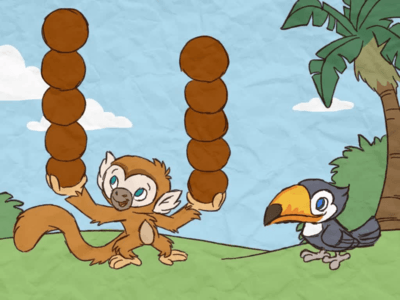Subtraction isn't sad -- you have to take some things away, but you get to share! Learn the basics of subtracting in this sweet song about friendship.

## Treasure Chest Subtraction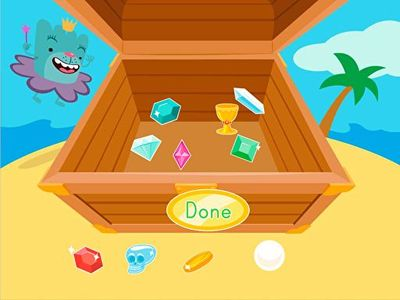That Tutu sure is generous. She's willing to share her treasure...but only if your kid listens closely and gets his subtraction facts right! First Tutu will tell him how many piece of treasure he can take out of his chest, and then it's up to him to pick out some treasure. If he successfully takes away the right number of items, he'll get to keep the booty for himself in this game that focuses on basic subtraction skills.

## Penelope's Flower Subtraction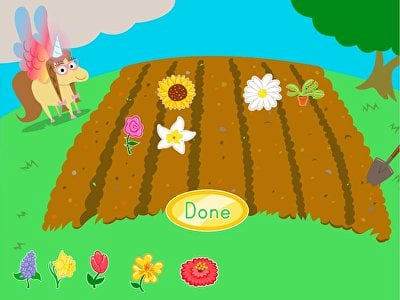Penelope's garden is blooming with flowers, and she needs help picking some. In this interactive subtraction game, kids must pluck the correct amount of flowers to solve subtraction problems in a concrete way. Great for visualizing subtraction, this manipulatives-based game is a perfect way for children to move from concrete to abstract subtraction.

## Manny's Bananas Story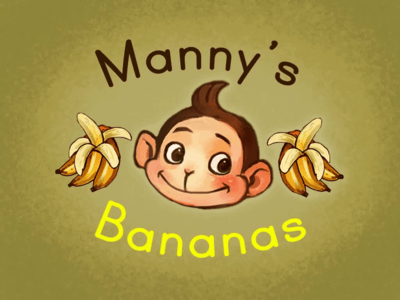Manny loves bananas, but he also loves sharing. Learn subtraction with the help of a generous monkey named Manny.

## Subtraction Quiz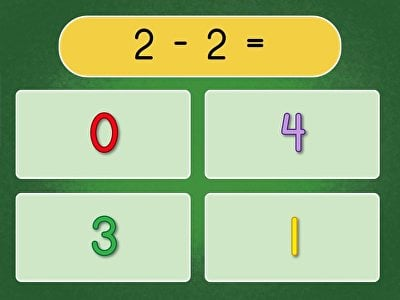Put down the manipulatives—it's time for mental subtraction! In this colorful quiz game, kids must answer simple subtraction problems without objects to help them. Perfect for early learners who are transitioning from concrete subtraction to abstract subtraction, this colorful math quiz helps children get the hang of mental subtraction within 10.

## Muggo's Subtraction Puzzle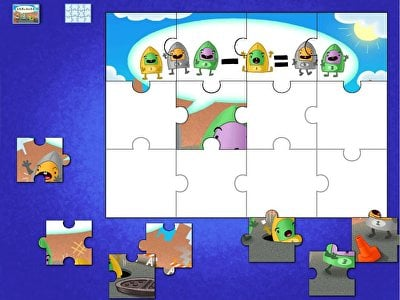Three Muggos strolled down the street, and one fell into a manhole! How many Muggos are left over? Perfect for early math learners, this simple subtraction problem in this silly puzzle game shows an image that clearly represents the equation 3 - 1 = 2. Seeing the equation drawn out will help children become more comfortable drawing out additional equations, which is a very effective strategy for learning how to subtract.

## Quiz: Subtraction Within 10In this game, kids must test their subtraction skills without the aid of visuals. They must use their mental math skills to get the hang of subtracting numbers within 10. Great for early learners to get a hang of simple single-digit subtraction!

## Match: Subtraction Facts Within 5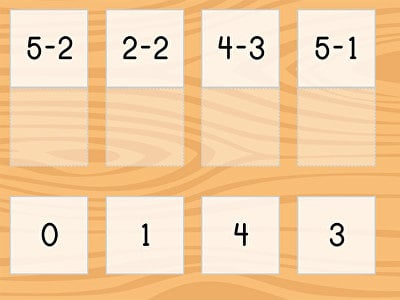Help your kindergartener hone their foundational subtraction skills with Match: Subtraction Facts Within 5. In this mental math matching challenge, players will try to match each subtraction equation to its answer. This game helps young learners get comfortable with the process of subtraction by focusing on subtraction within 5, building up their confidence before moving on to larger numbers.

## Ski Racer: Subtraction Patterns of 1 Less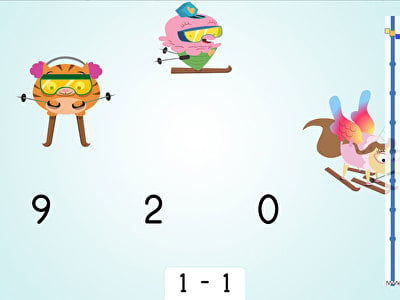Take 1 away in this online math activity. Made for students in preschool and kindergarten, this digital game challenges students to practice simple subtraction with single-digit numbers. The exercise Subtraction: Patterns of 1 Less showcases students’ ability to subtract the number 1 in a fun and interactive way. It also introduces a simple math pattern they can use on their own!